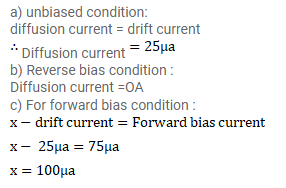# When a p-n junction is reverse-biasedn the current becomes almost constant

Question:

When a p-n junction is reverse-biasedn the current becomes almost constant at $25 \mu \mathrm{A}$. When it is forward-biased at $200 \mathrm{mV}$, a current of $75 \mu \mathrm{A}$ is obtained. Find the magnitude of diffusion current when the diode is

(a) unbiased,

(b) reverse-baised at $200 \mathrm{mV}$ and

(c) forward-baised at $200 \mathrm{mV}$.

Solution: